# International Economics Study Set 8

## Quiz 7 : Firms in the Global Economy: Export Decisions, Outsourcing, and Multinational EnterprisesLooking for Economics Homework Help?

## Quiz 7 :Firms in the Global Economy: Export Decisions, Outsourcing, and Multinational Enterprises

Question TypeIn perfect competition, firms set price equal to marginal cost. Why can't firms do this when there are internal economies of scale?
Free
Essay

Internal economies of scale imply that as output increases, the average cost of production falls i.e. average cost curve slopes downwards.
Whenever average cost is decreasing, the marginal cost will be less than the average cost because average cost includes the cost of those initial units that were produced at higher unit costs.
So, the marginal cost curve lies below the average cost curve. In this situation, if a firm sets its price equal to marginal cost (the cost of producing one extra unit of output), the average cost will be greater than the price; thus the concerned firm incur losses. The firms cannot sustain these losses and has to shut down in the long run.
Represent the graphs showing the price determination under perfect competition and internal economies as follows: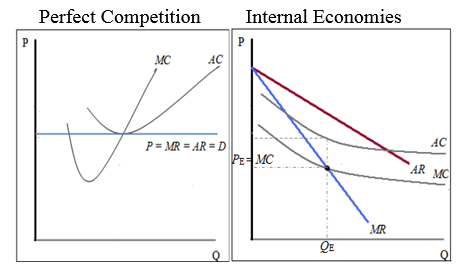In figure (a)firm under perfect competition sets the price equal to marginal cost where it is also equal to average cost, marginal revenue and average revenue. While, in the figure (b), firm under internal economies sets the price equal to marginal cost, so, the average cost being greater than the marginal cost, will incur losses.
Thus, firms can't set price equal to marginal cost when there are internal economies of scale because they incur losses.

TagsSuppose the two countries we considered in the numerical were to integrate their automobile market with a third country, which has an annual market for 3.75 million automobiles. Find the number of firms, the output per firm, and the price per automobile in the new integrated market after trade.
Free
Essay

If the two countries integrate their automobile industry with a third country, the number of firms, output per firm and the price per automobile is calculated as shown below;
The relation between average cost fixed cost and marginal cost is given by the below expression.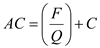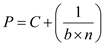Where,
Average Cost is represented as AC ,
Fixed Cost is represented as F ,
Marginal Cost is represented as C ,
Output of the firm is represented as Q,
Price is represented as P ,
Number of firms is represented as n ,
Constant (responsiveness of a firm's sales to its price)is represented as b ,
Calculate number of firms:
Since average cost per unit is same as the price, equate the average cost with price;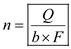However, S gives total output of the industry and it is equal to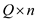as shown below;Total output S is equal to the total market of the three countries;Therefore, total market output of the three countries is 6.25 million.
Furthermore;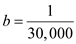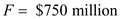Substitute S , b , and F value into equation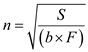:However, fraction of 15.8 firms does not exist, there will be 15 firms in the automobile industry (not 16)as the 16 th firm cannot cover its average cost or its average cost is higher than the market price of the industry.
Calculate Output per firm:Therefore, output per firm is 41,666 units.
Calculate price per automobile: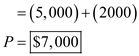Therefore, price per automobile is $7,000. Therefore, the total number of firms is 15, output per firm is 41,666 units, and the equilibrium price charged is$7,000.

TagsSuppose that fixed costs for a firm in the automobile industry (start-up costs of factories, capital equipment, and so on) are $5 billion and that variable costs are equal to$17,000 per finished automobile. Because more firms increase competition in the market, the market price falls as more firms enter an automobile market, or specifically, P = 17,000 + (150/ n ), where n represents the number of firms in a market. Assume that the initial size of the U.S. and the European automobile markets are 300 million and 533 million people, respectively. a. Calculate the equilibrium number of firms in the U.S. and European automobile markets without trade. b. What is the equilibrium price of automobiles in the United States and Europe if the automobile industry is closed to foreign trade? c. Now suppose the United States decides on free trade in automobiles with Europe. The trade agreement with the Europeans adds 533 million consumers to the automobile market, in addition to the 300 million in the United States. How many automobile firms will there be in the United States and Europe combined? What will be the new equilibrium price of automobiles? d. Why are prices in the United States different in (c) and (b)? Are consumers better off with free trade? In what ways?
Free
Essay

(a)It is given that P = 17,000 + (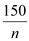), where P is price and n is the numbers of firms.Now, as we know that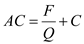=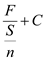(for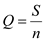)=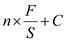Where, AC = average cost F = fixed cost Q = output of firm C = variable cost (marginal cost depends on variable cost and is independent of fixed cost)S = each country's market for the automobile sector
Since average cost per unit is same as the price; AC = P ,
17,000 + ()=..... (1)Now, the value of S in United States is 300 million. So, Substituting the values for S , F , and C in equation (1)we get the value of n for United States; n = 3. Similarly the value for S in Europe is 533 million. Substituting the required values in equation (1), we get the value of n for Europe; n = 4.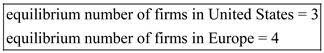(b)Substituting the value for n in the equation P = 17,000 + ()we get the value of P for United States; P US = 17,000+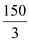=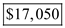Similarly, substituting the value for n in the equation P = 17,000 + ()we get the value of P for Europe; P E = 17,000 +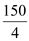=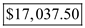(c)The combined value of S in United States and Europe is 833 million (300 million+533 million). Substituting the values for S , F , and C in equation (1)we get the value of n for both United States and Europe; n = 5.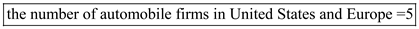Now, substituting the value for n in the equation P = 17,000 + ()we get the value of P for United States and Europe; P = 17,000 +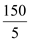= 17,030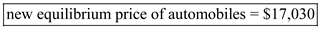(d)In both countries, the price of automobiles has fallen to $17,030 per automobile due to free trade. So consumers have to pay less for a purchase of an automobile after trade is opened. Not only this, consumers in each country have more variety of brands available after trade. In United States, the number of brands increases from three to five while that in Europe increases from four to five. Thus consumers in both countries are better off with trade. TagsGo back to the model with firm performance differences in a single integrated market Now assume a new technology becomes available. Any firm can adopt the new technology, but its use requires an additional fixed-cost investment. The benefit of the new technology is that it reduces a firm's marginal cost of production by a given amount. a. Could it be profit maximizing for some firms to adopt the new technology but not profit maximizing for other firms to adopt that same technology? Which firms would choose to adopt the new technology? How would they be different from the firms that choose not to adopt it? b. Now assume there are also trade costs. In the new equilibrium with both trade costs and technology adoption, firms decide whether to export and also whether to adopt the new technology. Would exporting firms be more or less likely to adopt the new technology relative to nonexporters? Why? Essay Answer: TagsIn the chapter, we described a situation where dumping occurs between two symmetric countries. Briefly describe how things would change if the two countries had different sizes. a. How would the number of firms competing in a particular market affect the likelihood that an exporter to that market would be accused of dumping? (Assume the likelihood of a dumping accusation is related to the firm's price difference between its domestic price and its export price: the higher the price difference, the more likely the dumping accusation.) b. Would a firm from a small country be more or less likely to be accused of dumping when it exports to a large country (relative to a firm from the large country exporting to the small country)? Essay Answer: TagsWhich of the following are direct foreign investments? a. A Saudi businessman buys$10 million of IBM stock. B) The same businessman buys a New York apartment building. C) A French company merges with an American company; stockholders in the U.S. company exchange their stock for shares in the French firm. D) An Italian firm builds a plant in Russia and manages the plant as a contractor to the Russian government.
Multiple Choice
TagsFor each of the following, specify whether the foreign direct investment is horizontal or vertical; in addition, describe whether that investment represents an FDI inflow or outflow from the countries that are mentioned. a. McDonald's (a U.S. multinational) opens up and operates new restaurants in Europe. b. Total (a French oil multinational) buys ownership and exploration rights to oil fields in Cameroon. c. Volkswagen (a German multinational auto producer) opens some new dealerships in the United States. (Note that, at this time, Volkswagen does not produce any cars in the United States.) d. Nestlé (a Swiss multinational producer of foods and drinks) builds a new production factory in Bulgaria to produce Kit Kat chocolate bars. (Kit Kat bars are produced by Nestlé in 17 countries around the world.)
Essay
TagsIf there are internal economies of scale, why would it ever make sense for a firm to produce the same good in more than one production facility?
Essay